# The Case for the Hypergeometrical Universe

## I Called Their Mothers Fat!!! by Marco Pereira on iBooks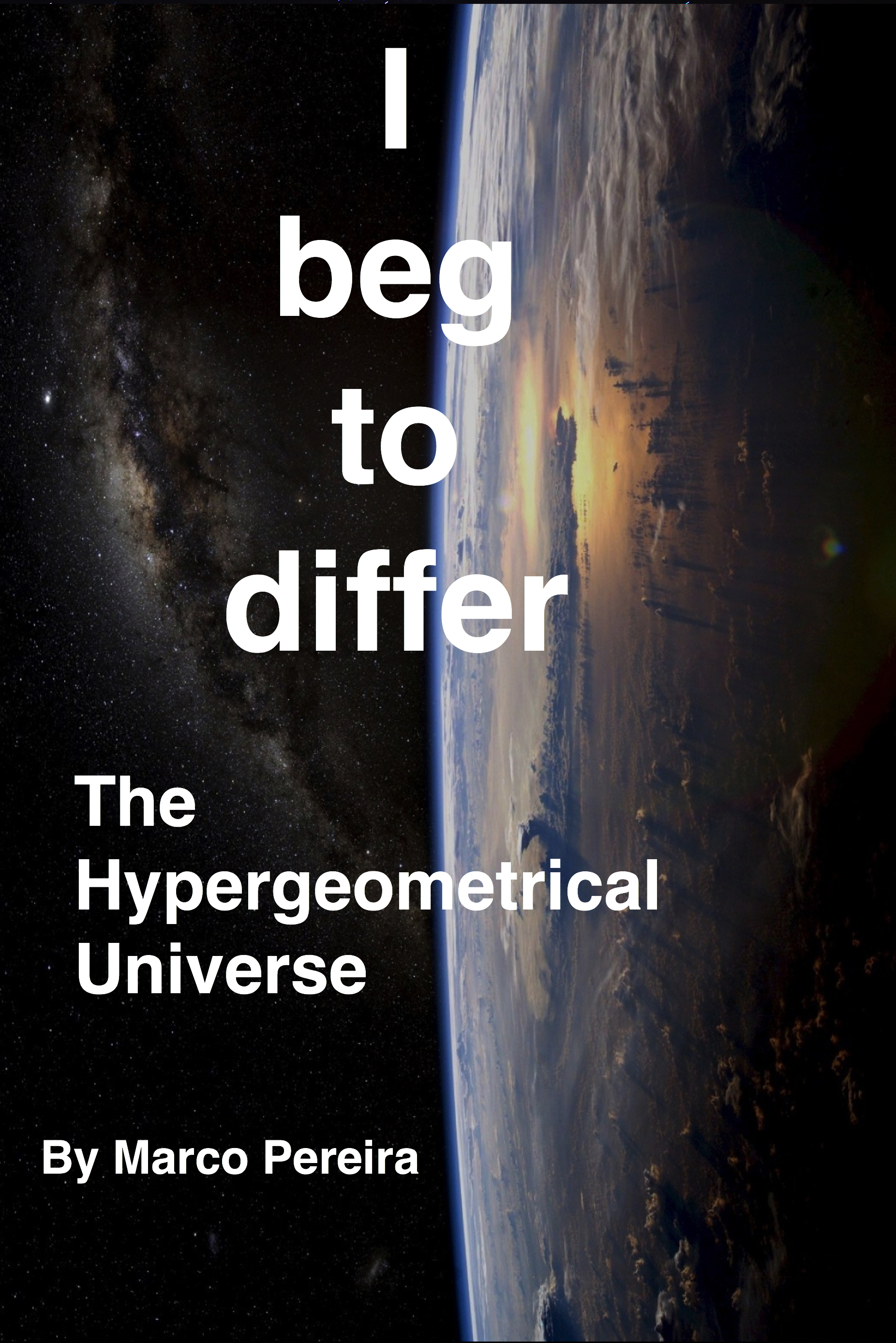# First Things First:

Luckly, albeit forgotten in the fog of time, the greatest people in Science aggreed with me.  Albert Einstein became a pariah (figure of speech - I read dinosaur in the past )... as soon as he delivered his 1915 General Relativity paper.  Not among the masses but among snide scientists.  He created Quantum Mechanics with the Photoelectric Effect paper, but he couldn't come to accept Quantum Mechanics and he couldn't figure out what was the hidden variable that would make the Universe return to fit his Classical and Sensible view....:)

The hidden variable was actually a hidden topology and a new model for matter - both which I proposed in my work.

Later, Feynman was horrified with infinities, renormalization, the particle Zoo...I am sure he was also horrified with the patchwork of Physics that followed.  If you cannot find a quark by itself, you create a glue to glue together the theory.  If your self-energy blows, renormalize - call it zero or one or anything.

Any process your don't understand, just create a new boson. There should be a loose Boson in the Particle Zoo.

The things got really worse.  Hubble discovered that the Universe was expanding which prompted Guth to cough up some inflation to patch things up again.  Higgs kicked the ball and pulled another Boson from the Particle Zoo to explain away mass.

Physics is now a fully grown edifice.. Complex, difficult to understand to the average Joe...:)

Anyone one of those really great people I mentioned (Einstein, Feynman) would agree with me that there was something stinking on that beautiful edifice.

## Let's look into the reasons for departure from Common Sense::

#### Dilaton Field and Pilot Wave Theory (de Broglie-Bohm Theory).

Michio Kaku has been seen stating with pride that the Universe is not explorable by Common Sense...  This is a common statement that I am sure Einsten suffered a lot while hearing it.

Reality is that the Universe is not accessible to our current Common Sense (prior to my theory).

For example, de Broglie - the Father of Matter Waves joined Bohm to create a theory where those waves were called Pilot Waves and had a physical meaning (independent upon the particle that create it).  In their theory, the pilot wave would pass through the two slits, while the electron would just pass through one. That is common sense, which Michio Kaku denied to be correct.

de Broglie-Bohm theory was only able to come up with a free range particle equation of motion (particle without a potential).$\frac{d \mathbf{Q}}{dt} (t) = \frac{\hbar}{m} \operatorname{Im} \left(\frac{\nabla \psi}{\psi} \right) (\mathbf{Q}, t)$.

The main reason this theory failed is because it didn't have an equation of motion that included a potential.  The pilot wave wasn't properly characterized and the other forces were not comparable (didn't have a dilaton field representation).

At first glance, it would seem that my de Broglie dilaton waves are the same thing as wavefunction.  That is not true.  My theory is not a rediscovery of de Broglie-Bohm theory.  Only people who is incapable of understanding details in both theories would conclude that.

The dilaton field I proposed and calculated strength is created by dilators (particles). The dilator will interact with the difracted dilaton field within the double-slit experiment and deposit itself  as an interferogram. This is not the same as saying the the particle is a wave and with a delocalized nature.

The fact that my theory at its simplest representation is two-dimensional XR (the forces are derived from two dimensional waves) indicates that each particle follows its path and it is localized.  The dilaton field from many particles would scramble trajectories and particle deposition would showcase a wavefunction interferometric profile (not unlike a single particle self-interferogram).

Dilators interact with self and mutual dilaton fields while they move in the 4D spatial manifold. The loci where they land within the 3D hyperspherical Universe maps to the Quantum Mechanical probability density or Wavefunction#* Wavefunction - (# is the Hermitian Conjugate).

Within the de Broglie-Bohm argument there is a non-locallity aspect. Not unlike Einstein, I don't see the need for nonlocallity considerations to satisfy Bell's Inequality. Communication of information related to spin can have speeds that dwarf the speed of light.  In my theory, the speed of light is SQRT*c and light is a spatial modulation on the top of a very fine dilaton wave.

t11/c= 6.39621*10-25seconds

is the time to traverse a de-Broglie step of λ1= 0.191753 femtometers

The grid wavelength for gravitation is the 0.29 Compton wavelength of two Hydrogen atoms. The basic unit for Gravitation is one Hydrogen atom, which looks like two hydrogen atoms if one considers the cohrences in four spatial dimensions.  The Gravitational step is what is being described  above.

The grid wavelength for electromagnetism is the 0.29 Compton wavelength of an Hydrogen atom.. 0.29 accounts for the different frequency associated with hypervolumetric dilaton waves ..traveling at the speed of light... about 1/4 of the size of a proton.  The Universe step is 12.8 E-25 seconds for Electromagnetic Fundamental Dilator...quite fast...These features are undetectable by experiments since any experiment only observes interaction and interaction is time-quantized.

The obvious positive side of this wavefunction interpretation is the solution to the wavefunction collapse problem. If a place an electron on a box and make a mesurement, the electron which could be any place within the box, suddenly appears in a single place.... Einstein hated this since it would mean faster than the speed of light communication.  Another example. Let's say that there was a photon that left a star 15 billion light years away and arrive at your eyes today.  The photon was delocalized, traveling in a spherical wavefront 15 billion light years radius of curvature.  As soon as you look at it, that wavefunction collapses and the electron is localized.  I don't need to tell you that that there is a problem here.

### Expanding Universe and Inflation:

Of course, after Hubble discovered Universe expansion it didn't take too long for Dr Guth to propose the Inflation Theory. The reason is the problematic isotropy of a 3D Universe model.  In an Universe where there is only 3 non-compact spatial dimensions (no matter how many compact (infinitesimal) dimensions string theorists propose) a 15 Billion light years old Universe has at leas 30 billion light years dimension.

Since there is an UNIFORM Microwave Cosmic Background, there has to be some way to the initial Black-Body radiation to be thermalized before the Universe inflates to at least twice the appropriate size.  That is what I call a Rube Goldeberg apparatus created by Dr. Guth.

Occam Razor would support that:

• add an extra non-compact spatial dimension
• place the 3D Universe in a 3D Hypersurface
• place it in motion at the speed of light radially (that doesn't offend Restrict Relativity since that theory is only valid for 4D Spacetime).
• make the speed of light be Sqrtc (this doesn't collide with any speed of light measurement)

if you can come up, as I did, with any other good reason why this topology is ideal, that is better.

In my case,  I added a new model for matter (it doesn't have quarks so it doesn't need glue nor gluons).

This is my case for the topology and my initial case for the Hypergeometrical Universe Standard Model.

### Review of the Laws of Dynamics:

Laws of Dynamics are the laws relating force and acceleration (Newton's 4th Law), constant motion (Newton's First Law).

I tried to answer the question: What is the minimum I need to know to say something about motion?

Everyone would say that if you fall into a Black-Hole you become part of it. Black-Holes are metric deformations (singularities).  The obvious thing to do is to create a model for matter that is also related to metric deformations.  The next question: Do I need to know anything about the metric deformation potential or can I just use the deformation "stationary" states (particles).  I decided to just use the states we know as masses and think a little.

Using space and space deformations as the basis for the Hypergeometrial Physics implies that one should use a Stress-Strain space deformation model. Strain maps to mass and stress to force.

Newton's equations maps easily to Stress-Strain model.

Newton's and Gauss's Laws have poles at zero distances.  That is not a problem when dealing with macroscopic bodies, but becomes a huge problem with dealing with fundamental particles. The electron-self energy blows to infinite.

Since now particles are dilators (coherences between statitionary metric deformation states spinning within the 4D spatial manifold while traveling at the speed of light radially) interacting through dilaton fields (propagating metric waves or deformations).

If dilators are interacting through waves (dilatons), the obvious Quantum Lagrangin Principle is just a minimum work principle. Dilators should always be in phase with the local dilaton field.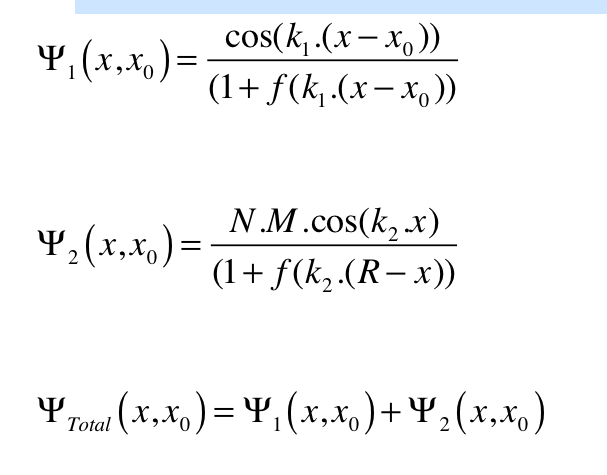Here we have two dilators (dilator 2 is modeled as a Kg of dilators and dilator 1 is a probe dilator for us to calculate the force acting upon one dilator).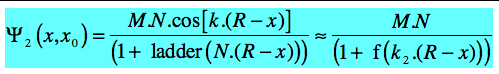Since we are using a Kg of dilators, there is a big number of them...:) that means that the numerator oscillates very fast, so one can always find another maximum without going too far, so the cosine is mapped to 1 - all the time.  This is done to derive Newton's Gravitational Law, Gauss Electrostatic Law and Biot-Savart Law.

The M is there to map gravitation and anti-gravitation. N is the number of dilators.

Now just that the derivative with respect to x and solve it. This would give the shift x where the dilator would land after a de Broglie step expansion of the Universe (I will explain this quantization soon).  A shift defines a change in angle of the local metric, that new angle is where the K-vector of the dilator is poised afterwards. So at the first step a shift means a change in velocity.  The second step will see a equal shift taken from the new K-vector direction, so it will represent an acceleration.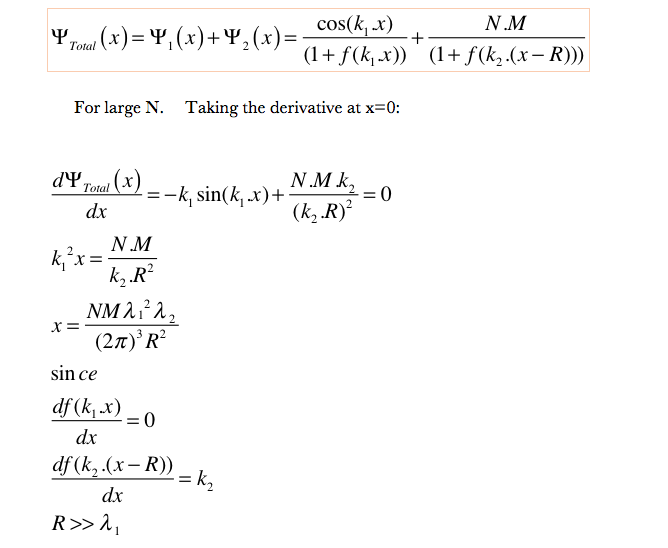As you can see, one can calculate the displacement x using high school math (first order derivative and first order taylor expansion of a sine function).

Once you have acceleration, you can just multiply by the number of dilators in a Kg to find out the force between two kg (Newton's Gravitational Law).  Or scale this by the number of dilators in a Coulomb to get Gauss Law.

### How come both Gauss' Law and Newton's Law yields the same value for x????

The trick of the tail is that Gravitation is the result of neutral combination of dilators. There is such a thing called Van der Waals force which is a result of fluctuations and correlation between charge fluctuations.  This is an always attractive force between neutral molecules or moyeties. The dilators also fluctuate as they move around at much higher speed that charge visibly fluctuates.  This is also the reason for Gravitational Attraction - and this is an OBVIOUS conclusion.

Not unlike Van der Waals, this force is smaller that naked charge interaction or electrostatics.  In the case of Gravitation, the nature of the shielding is much more effective and have higher frequency.  If you thing about the wavefunction as the loci of dilator footprints, you can think that Van der Walls is created by fluctuations on that wavefunction.  Gravitation is created because of the extremely fast fluctuations of dilator position (dilator jumps around the wavefunction at each de Broglie step), so dilator fluctuations is much faster and accomodating of dilator interaction and thus much more effective in neutralizing interaction - thus creating a much weaker attraction!!!!!

The remaining dilaton field shoudl have zero effect, that is, the dilator would move to a position where it would satisfy the Quantum Lagrangian Principle, that is, where the total dilaton field derivative is zero, but its k-vector would remain pointing radially (relaxed local metric). This last distinction explains the difference in strenght between Gravitation and Electromagnetism.

In my theory, I used the observed Gravitational Constant to derive the natural frequency of the dilaton field. The metric is elastic and from its elasticity constant one can derive its natural frequency.

### Biot-Savart Law:

For the case of Biot-Savart Law derivation, I placed the large dilator (large number of dilator) at position   R/Sqrt] (1,1,1,0) to obtain an expresion that would depend on all cosine directors.  The calculation was similar to the uni-dimensional derivation. I use simple reasoning stating that the position x for dilator 1 would point along the direction where dilator 2 was dragging the metric, that is :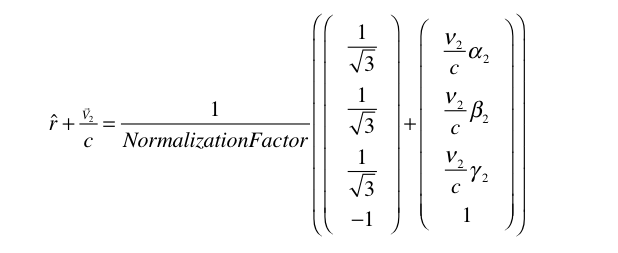for example the position of dilator 2 after R/c seconds is R/Sqrt + v2.alpha2*(R/c). If you divide that by R, you will be able to understand the direction vector I proposed.  Simple minded problem, although in four dimensions it becomes more challenging..:)

I almost forgot the normalization factor for that vector. K-vector is 2Pi/Lambda times something.  We want that something to be between zero and one.

Under those conditions, the normalization factor is: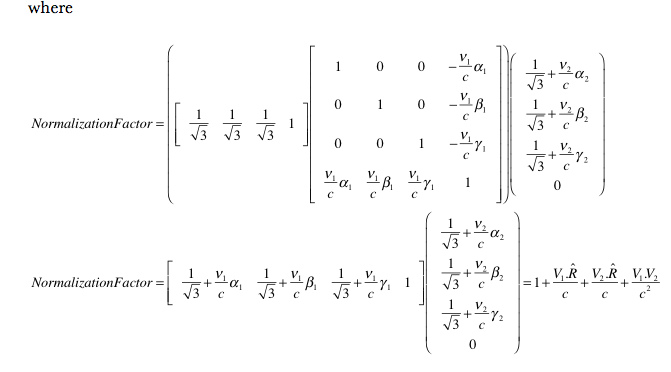After that I calculated x according to my simple math and corrected by the normalizaion factor to obtain this value (I called Delta here)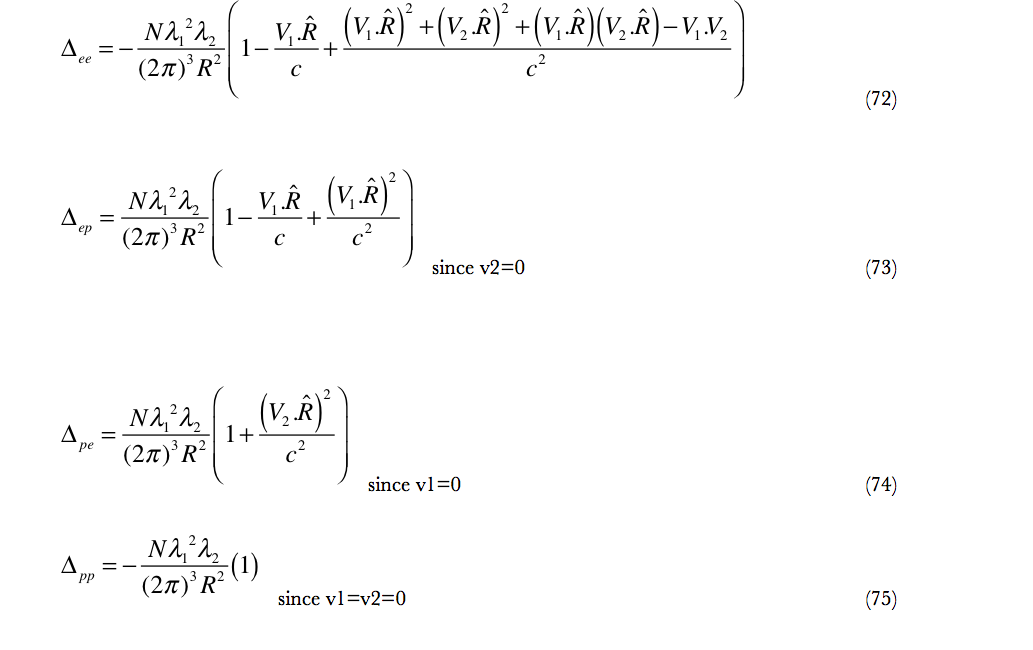I only obtained one value DeltaEE.  That is already a linearization for small velocities. I observed that for me to obtain the Biot-Savart Law, I had to consider all four contributions ee (eletron1-electron2 hole1-electron2 electron1-hole2 and hole1-hole2)

That is, the force between two wires carrying current depends not only on the electronic charges moving but also on the holes they leave behind.  This was never evident to me from reading Biot-Savart equation since that is a Natural Law, derived experimentally and not from first principles.

This means that my theory unveils something that other models do not. This is a very strong case for it to receive a critique and thoughful analysis - nothing else.  I called it the Theory of Everything because I did my personal critique and thoughtful analysis.  I also begged, pleaded with scientist to do the same and take a position since IDEAS ARE IMPORTANT!!!!

## The Fat Electron:

If you look what my electron model looks like, you will realize that it looks exactly the same as my proton, antiproton, and positron models.

## I beg to differ!!!

None of the experimental results would detect a shape shifting, spinning dilator from something static.  You just have to consider that interaction is proportional to the dilator state footprint on the 3D Universe and conclude that sideways dilators are skinny.

The states are shown below: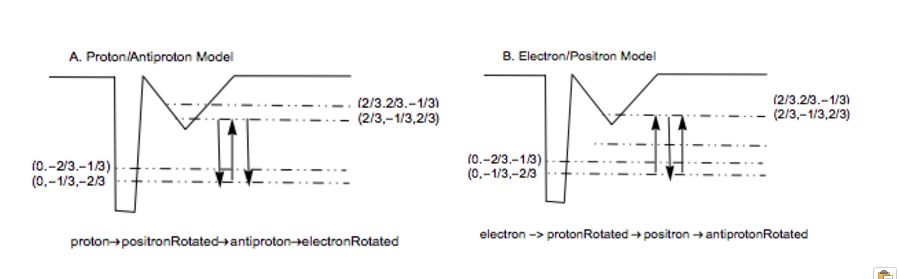I moved the proton state to the first excited state and place the electron into the lowest state - this was done because of their footprint. The lowest state would have a narrower wavefunction that an excited state. An interesting and surprising result.

The origin of the first arrow indicates state in phase with the Universe. The dilator tunnels between states and spins within a four-dimensional non-compact spatial manifold. This means that at every transition it goes in and out of visibility (interaction) with the 3D Universe.  This is the reason why the dilator can keep its character. The states represent "stationary" states of local metric deformation. I represent the Proton with a large stretch and an electron with a small compression. After 180 degrees (two tunnelings) these inverts. A proton becomes a large compression and an electron becomes a small stretch.

For a long time, I thought about the nature of these states. Are they truly 3D or the Universe has some thickness along the radial direction. In nature, very if any thing are truly thin..

In any event, since the charge of an electron is equal to the charge of a proton, their dilaton waves should be generated by all four phases of the dilator coherence. This means that Electromagnetism and Gravitation are the results of 4D volumetric modes.  de Broglie Field is dependent only upon the footprint of the dilators, thus it is a hypersuperficial mode.  Read more on the book.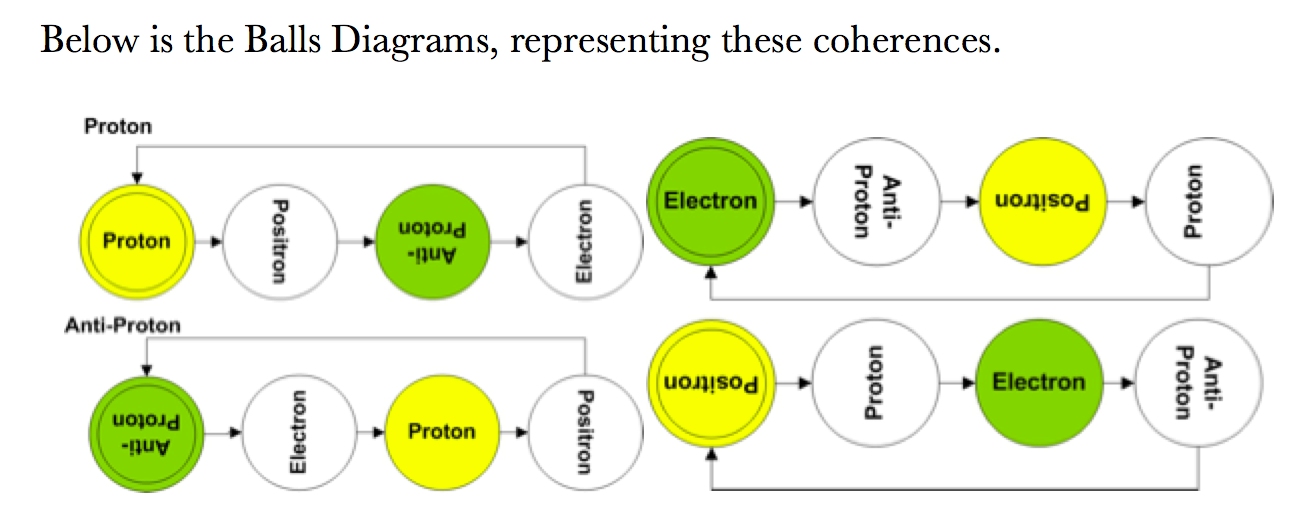Horizontal lettering means flush with the 3D Universe. Vertical lettering is perpendicular to the 3D Universe and thus invisible (non-interacting).  Albeit invisible, they represent changes in the local metric and contribute to hypervolumetric modes (Gravitation and Electromagnetism).

Below is a more complex coherence (Pion Minus):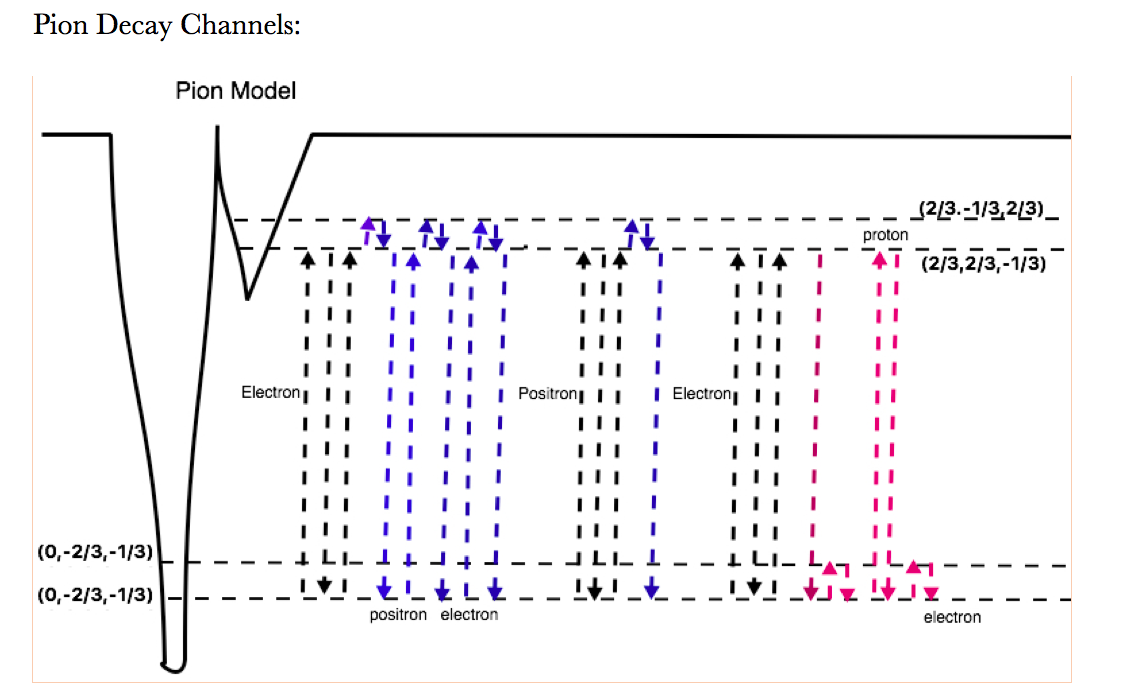You can see that a pion minus is composed of two electrons and a positron - plus two electro neutrinos and a muon neutrino.  Find them in the picture..:)

Since you can see all the phase of en electron dilator, the electron, the proton, positron and antiproton have all the same masses from the 4D perspective. They all weight the same - an Hydrogen Mass!!!!  That is relevant for strength comparison between the forces and also for obtaining the frequency of the different dilaton fields.  As expected, Electromagnetism has half the frequency of Gravitation and that can be easily calculated from my theory.  The frequency of a de Broglie dilator is m0.c^2/h...:0 as one would expect.

# Q.E.D.

I made my case for my theory and mentioned en passant that the current Physics Edifice is nicely decorated but sits on a Smoldering Pile of Trump.  The band-aids currently in use are terrible.... horrendous... offensive to a Physicist.  Mathematicians on the other hand, love decorating challenges... as long it looks good from the top, let's not look too close.

I try to make the point that my theory allows for a fresh look at the base where this nice Edifice sits.  I certainly don't envision the need to replace Schrodinger equation - it works and I am sure we would derived it using my fields.  The only difference is interpretation... Abandon the Copenhagen Interpretation... Forget about Particle-Wave duality and other nonsense....  The exercise of deriving the Schrodinger equation is worthwhile for the same reason the exercise of deriving the Biot-Savart Law was.  It might unveil something we didn't understand properly.  I don't need to tell you that Schrodinger equation wasn't derived.

Revise the Standard Model.... or at least give my model a shot.  I a sure you don't expect me to do all your work for free...:)

My electromagnetism equation might do wonders for EM Drive and your next trip to Saturn...as well as how to make a better magnetic bottle or a better tokamak...

Coherent nuclear fusion might be the way we will power our future electric or EM Drive airplanes.

There is a lot to be gained and very little to loose.

Engage with me if you have any questions.

I will make space for Big Wigs in my site to post their comments and my replies.   Just look on the top menu for BigWigs.. The initial ones will be the talking heads.... people who gets paid to disseminate Science (Neil deGrasse Tyson, Michio Kaku, Bryan Greene...).  They should disseminate my Science if it it is proper Science.

If you are a Big Wig and want to be part of the discussion, please sign up and leave a comment or send me an email directly and I will create a blog to place our discussion.

Cheers,

MP

Current rating: 5

### New Comment

required

required (not published)

optional

required